# SAT Math Multiple Choice Question 896: Answer and Explanation

### Test Information

Question: 896

11. Given that a ≠ ±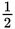, which of the following is equivalent to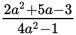?

• A.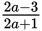• B.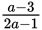• C.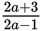• D.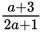Explanation:

D

Difficulty: Medium

Category: Passport to Advanced Math / Exponents

Strategic Advice: When dividing polynomials, you have two choices: factor and cancel, or long division. Choose whichever method you're more comfortable with.

Getting to the Answer: Factoring is most likely the faster route. The denominator is easier to factor, so start there: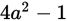is a difference of squares, which becomes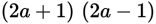. The expression in the numerator will factor into two binomials, one of which must be either 2a + 1 or 2a - 1 so that the fraction can be reduced. Using factoring by grouping, find the factors of 2(-3) = -6 that add up to +5, split the middle term using those numbers, and then factor by grouping: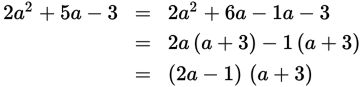The factor 2a - 1 appears in the numerator and the denominator, so cancel it to leave, which is (D).